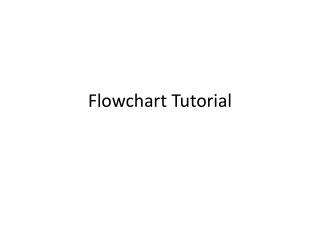DownloadDownload PresentationFlowchart Tutorial

# Flowchart Tutorial

Télécharger la présentation## Flowchart Tutorial

- - - - - - - - - - - - - - - - - - - - - - - - - - - E N D - - - - - - - - - - - - - - - - - - - - - - - - - - -
##### Presentation Transcript

1. Flowchart Tutorial

2. Example 1: Draw a flowchart to find the sum of first 50 natural numbers.

3. start SUM=0 N=0 N=N+1 SUM=SUM+N no IS N==50? yes Print SUM END

4. Example 2 Draw a flowchart to find the largest of three numbers A, B, and C.

5. START READ A,B,C yes IS B >C ? no IS A>B ? yes IS A>C ? yes no no PRINT C PRINT A PRINT B END

6. Example 3 Draw a flowchart for program Find the difference and the division of two numbers and display the result.

7. START READ N1 , N2 D=N1-N2 V=N1/N2 PRINT D , V STOP

8. Example 4 Draw a flowchart for program Print the first 10 natural number

9. start N=1 Print N N=N+1 yes while N <= 10 no END

10. Example 6 Draw a flowchart for program Calculate the student grade ( A,B,C,D,F)